# Measuring Center

• Median

• The middle number

• The median is resistant to outliers

• Mean

• The average number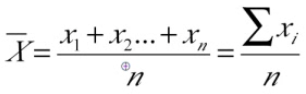• Calculation

• STAT + CALC + 1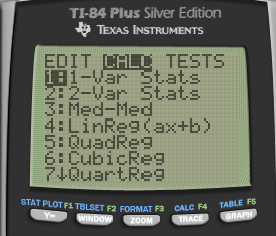• 2ND + 1 (L1)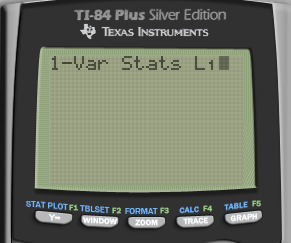• Enter# Measuring Position

• Percentiles

• Percentage of observations your value is above

• 30th percentile is the value below which 30 percent of the observations may be found

• Take the average if there are two values

• Quartiles

• Q1 = first quartile = 25th percentile

• Q2 = median = 50 th percentile

• Q3 = third quartile = 75th percentile

• Range

• Highest - Lowest
• IQR (Interquartile Range)

• Q3 - Q1
• Variance / Standard Deviation• Use Sx in the TI-84 calculator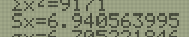# Outliers

• 1.5 * IQR rule

• Q1 - 1.5 * IQR = Bottom fence

• Q3 + 1.5 * IQR = Upper fence

• Outside the "fence" = Outlier

• Example# Boxplots

• Graph of the "5-number Summary"

• Min Q1 Median Q3 Max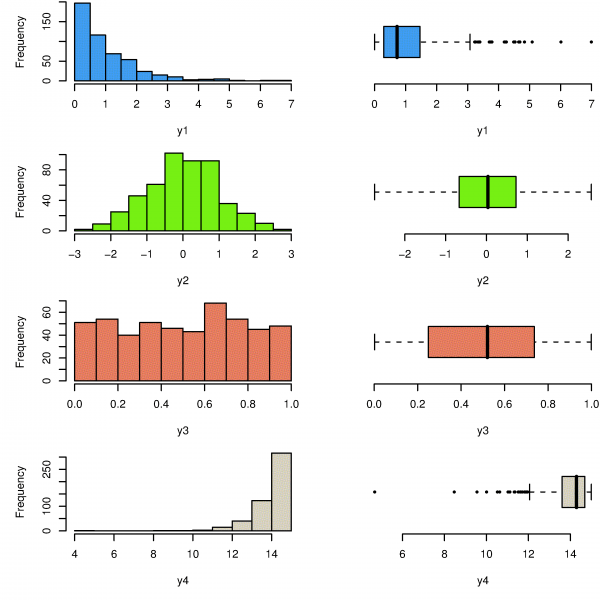• Example

• 18, 18, 17, 27, 22, 19, 20, 21, 18, 35, 32, 40

• Min: 17

• Q1: 18

• Median: 20.5

• Q3: 29.5

• Max: 40• Calculator

• Boxplot• Modified Boxplot# SOCS

• Shape

• Skewed left/right
• Outlier

• Q1 - 1.5 * IQR

• Q3 + 1.5 * IQR

• Center

• Mean or Median

• SD or IQR
• Example

• 18, 18, 17, 21, 26, 40, 23, 27, 22, 19, 20, 21, 18, 35, 32

• Skewed right

• No outliers

• Centered at a median of 20.5

• A spread of IQR = 11.5

# The Effect of Changing Units on Summary Measures

• Linear Transformations

• Xnew = A + B * Xold

• A: only affect mean and median

• B: affect all

# Examples• Construct a modified boxplot for this data set

• Min = 56

• Q1 = 66

• Med = 72

• Q3 = 77

• Max = 100

• IQR = Q3 - Q1 = 11

• IQR * 1.5= 16.5

• Bottom fence = Q1 - IQR * 1.5 = 49.5

• Upper fence = Q3 + IQR * 1.5 = 93.5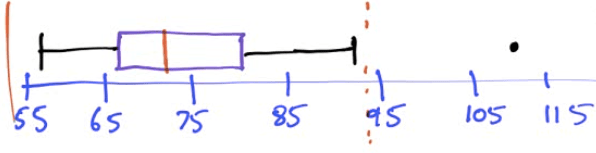• Describe the distribution of test scores

• Symmetric

• One outlier at 110

• Centered at a median of 72

• With a spread of IQR of 11